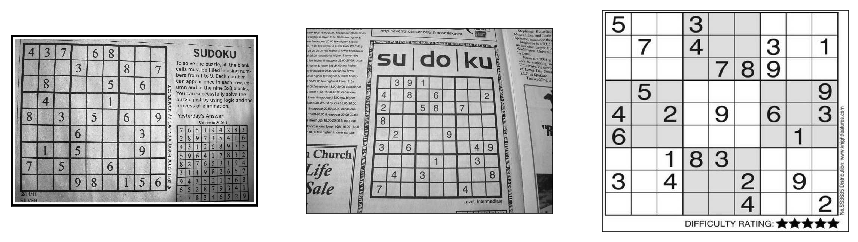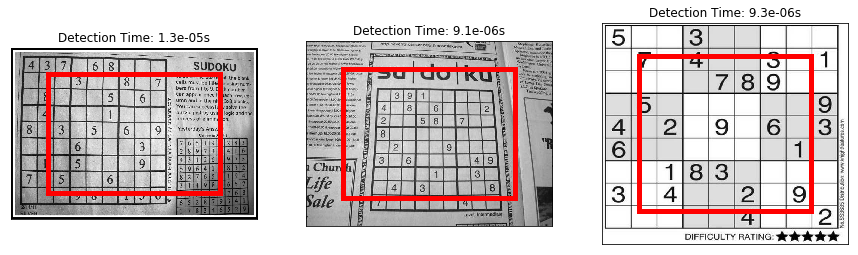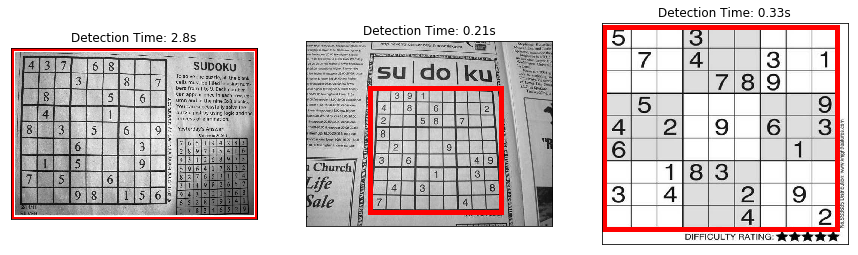Sudoku is a very interesting puzzle, and it is very easy to get familiar with the rules of the game. I always like to compare sudoku with number theory, they are easy to be understood but can be hard to solve. Fermat's Last Theorem, an easily understood problem even by middle school students, took mathematians 358 years to solve it completely. Solving sudoku puzzles can take even longer: it is proven that solving sudoku puzzels is an NP-complete problem! However, classical 9x9 sudoku puzzles are still feasible using backtracking algorithm. We are not going to talk about how to solve sudoku puzzles here though, we are trying to sovle another problem: detect grids in images containing sudoku puzzles.

To make the problem more concrete, let's make some assumption on the image that contains a sudoku puzzle:

1. The image only contains one sudoku puzzle
2. The sudoku puzzle is the largest block in the image
3. There is no/little distorsion/rotation of the sudoku puzzle grid

Also in this article, we focus on only simple solutions without heavy image processing. We will use Pillow to read images and convert them to gray scale images, and we use Numpy to manipulate images as arrays. For visualization, we use Matplotlib.

```import sys
py = 'Python ' + '.'.join(map(str, sys.version_info[:3]))
print('Jupyter notebook with kernel: {}'.format(py))

import time
from PIL import Image
import numpy as np
import matplotlib.pyplot as plt
```
```Jupyter notebook with kernel: Python 3.7.0
```

We will first load some images of sudoku puzzles that we are going use througout the article. All images are collected from the internet. I put a copy of these images here: sudoku0.jpg, sudoku1.jpg, sudoku2.jpg

```images = []
for n in range(3):
img = Image.open(f'sudoku/sudoku{n}.jpg')
# convert image to gray scale
img = img.convert('L')
images.append(np.array(img))

plt.figure(figsize=(15, 30))
for n in range(3):
plt.subplot(1, 3, n+1)
plt.imshow(images[n], cmap='gray')
plt.xticks([])
plt.yticks([])
plt.show()
```### 0. Setup

In this section, we are going to setup some codes for later use. We will create a base class `GridDetector`: it will assume the middle part of the image is the grid of the sudoku puzzle. We will subclass `GridDetector` to implement better solutions later. We will also define a function `check(DetectorClass, images)` to visually show the results of our grid detectors.

```class GridDetector:

def __init__(self, image):
self.image = image
self.row, self.col = self.image.shape

# coords is a tuple (x1, y1, x2, y2) representing the bounding
# box of the detected grid
self.coords = None

def grid(self):
'''Return a tuple (x1, y1, x2, y2) indicating the grid,
where (x1, y1) is the upper left corner of the grid,
and (x2, y2) is the lower right corner of the grid.

All subclass should implement this method.'''
if self.coords is not None:
return self.coords
x1 = int(self.col * 0.15)
y1 = int(self.row * 0.15)
x2 = int(self.col * 0.85)
y2 = int(self.row * 0.85)
self.coords = (x1, y1, x2, y2)
return (x1, y1, x2, y2)

def plot(self, plt):
plt.imshow(self.image, cmap='gray')
start = time.time()
x1, y1, x2, y2 = self.grid()
time_used = time.time() - start
plt.plot([x1, x1], [y1, y2], 'r-', lw=5)
plt.plot([x1, x2], [y2, y2], 'r-', lw=5)
plt.plot([x2, x2], [y1, y2], 'r-', lw=5)
plt.plot([x1, x2], [y1, y1], 'r-', lw=5)
plt.xticks([])
plt.yticks([])
plt.title(f'Detection Time: {time_used:.2}s')

def check(DetectorClass, images):
n = len(images)
plt.figure(figsize=(15, 30))
for i, image in enumerate(images):
plt.subplot(1, n, i+1)
DetectorClass(image).plot(plt)
plt.show()

check(GridDetector, images)
```### 1. Largest Black Connected Component

Our first idea makes use of the second assumption on the image: the sudoku puzzle is the largest block in the image. Recall that in a gray scale image, a pixel of value 0 is black and that of value 255 is white. However, the pixels forming the grid do not have to be of value 0 to look black. Indeed, we will treat pixels with value less than 80 black. We then use flood fill algorithm to find the largest black connected component, which we will assume to be the grid we are looking for.

```class LargestComponent(GridDetector):

threshold = 80

def grid(self):
if self.coords is not None:
return self.coords
visited = set()
for i in range(self.row):
for j in range(self.col):
if (i, j) in visited or self.image.item(i, j) >= self.threshold:
continue
x1, y1, x2, y2 = self._flood_fill(i, j, visited)
if self.coords is None:
self.coords = (x1, y1, x2, y2)
else:
a1, b1, a2, b2 = self.coords
if (x2 - x1) * (y2 - y1) > (a2 - a1) * (b2 - b1):
self.coords = (x1, y1, x2, y2)
return self.coords

def _flood_fill(self, i, j, visited):
r1 = r2 = i
c1 = c2 = j
stack = [(i, j)]
while stack:
r, c = stack.pop()
for nr, nc in [(r+1, c), (r-1, c), (r, c+1), (r, c-1)]:
if nr < 0 or nc < 0 or nr >= self.row or nc >= self.col:
continue
if (nr, nc) in visited or self.image.item(nr, nc) >= self.threshold:
continue
r1 = min(nr, r1)
r2 = max(nr, r2)
c1 = min(nc, c1)
c2 = max(nc, c2)
stack.append((nr, nc))```image0 = images[20:-20, 20:-20]